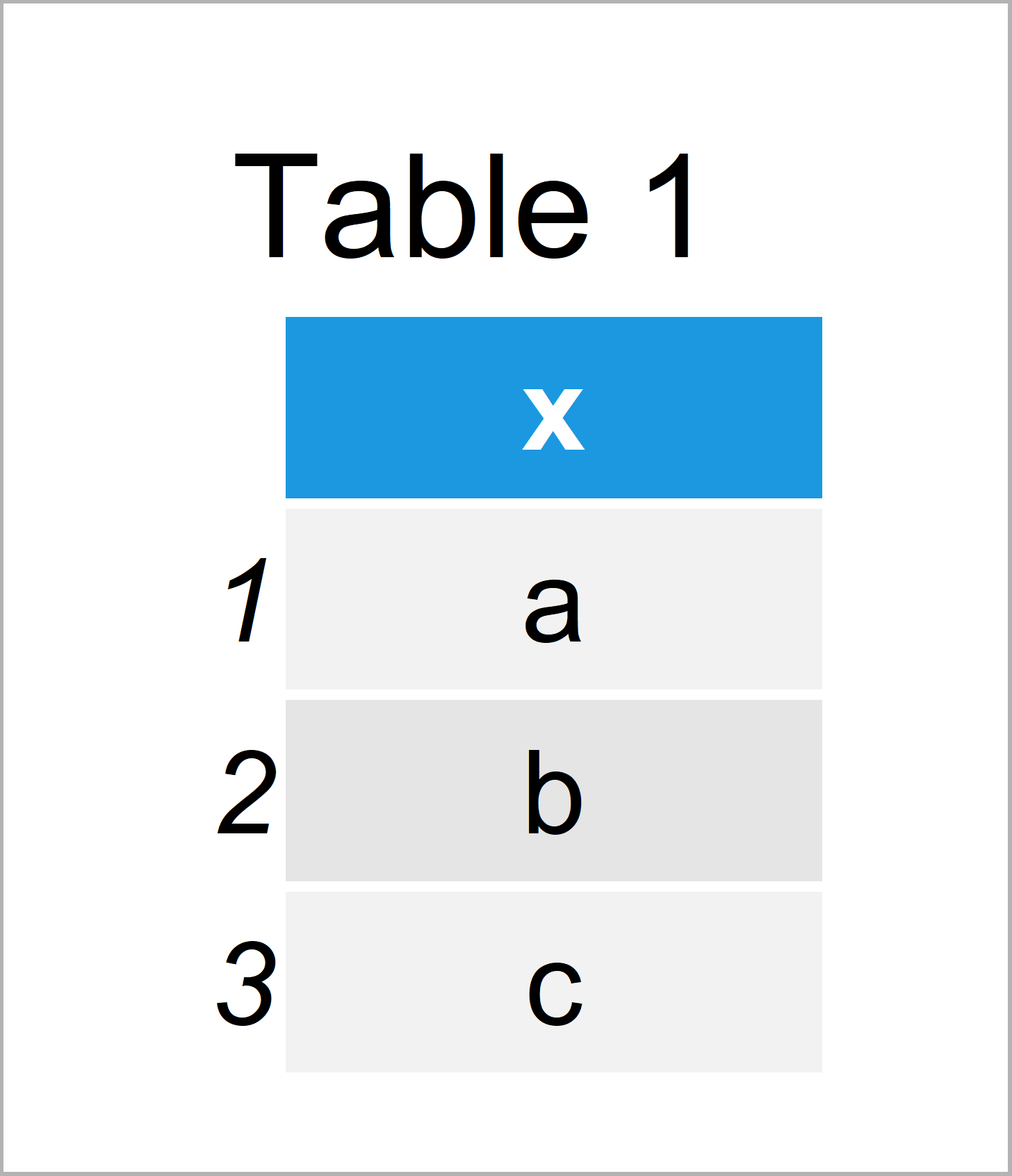# Extract Unique Values in R (3 Examples)

In this article you’ll learn how to select only unique values from a vector or data frame column in the R programming language.

The tutorial consists of this:

Let’s dive right into the examples…

## Creation of Exemplifying Data

We use the following data as basement for this R programming tutorial:

```x <- rep(letters[1:3], each = 3)    # Create example vector
x                                   # Print example vector
#  "a" "a" "a" "b" "b" "b" "c" "c" "c"```

The previous output of the RStudio console shows that our example vector consists of nine character elements. Each vector element exists three times.

## Example 1: Apply unique() Function to Select Unique Values

This example shows how to apply the unique function to select only unique values from our example vector.

Consider the following R code:

```unique(x)                           # Using unique function
#  "a" "b" "c"```

After executing the previous R syntax, the RStudio console returns a new vector showing the three unique values of our vector (i.e. a, b, and c).

## Example 2: Apply duplicated() Function to Select Unique Values

The following R syntax illustrates how to use the duplicated function to get all unique values from our vector:

```x[!duplicated(x)]                   # Using duplicated function
#  "a" "b" "c"```

The result is the same as in Example 1.

## Example 3: Apply distinct() Function of dplyr Package to Select Unique Values

It is also possible to use functions of the dplyr package to extract unique values.

We first need to install and load the dplyr package:

```install.packages("dplyr")           # Install dplyr package

Now, we can use the distinct function of the dplyr package in combination with the data.frame function to return unique values from our data:

`distinct(data.frame(x))             # Using distinct function`As shown in Table 1, we have created a data frame showing only unique values of our input vector x by running the previous R programming code.

Note that we had to convert our vector object to the data.frame class first, since the distinct function is not taking vector objects as input.

If you are looking for unique values in a data frame variable anyway, you don’t need to use the data.frame function as we did in this example.

## Video, Further Resources & Summary

Would you like to know more about unique values? Then I recommend watching the following video of my YouTube channel. In the video, I’m illustrating the R code of this article in the R programming language:

Please accept YouTube cookies to play this video. By accepting you will be accessing content from YouTube, a service provided by an external third party.If you accept this notice, your choice will be saved and the page will refresh.

In addition, you might want to read the other R programming articles of Statistics Globe. I have published numerous tutorials about related topics such as matrices, counting, and groups:

In this R article you have learned how to get unique values of an array or a data frame variable. If you have additional questions, please let me know in the comments section below.

Subscribe to the Statistics Globe Newsletter